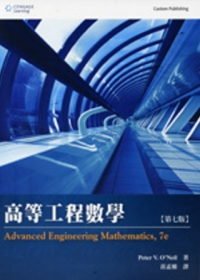{itemname} {itemname}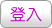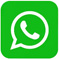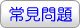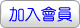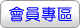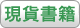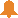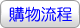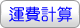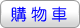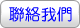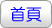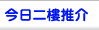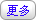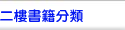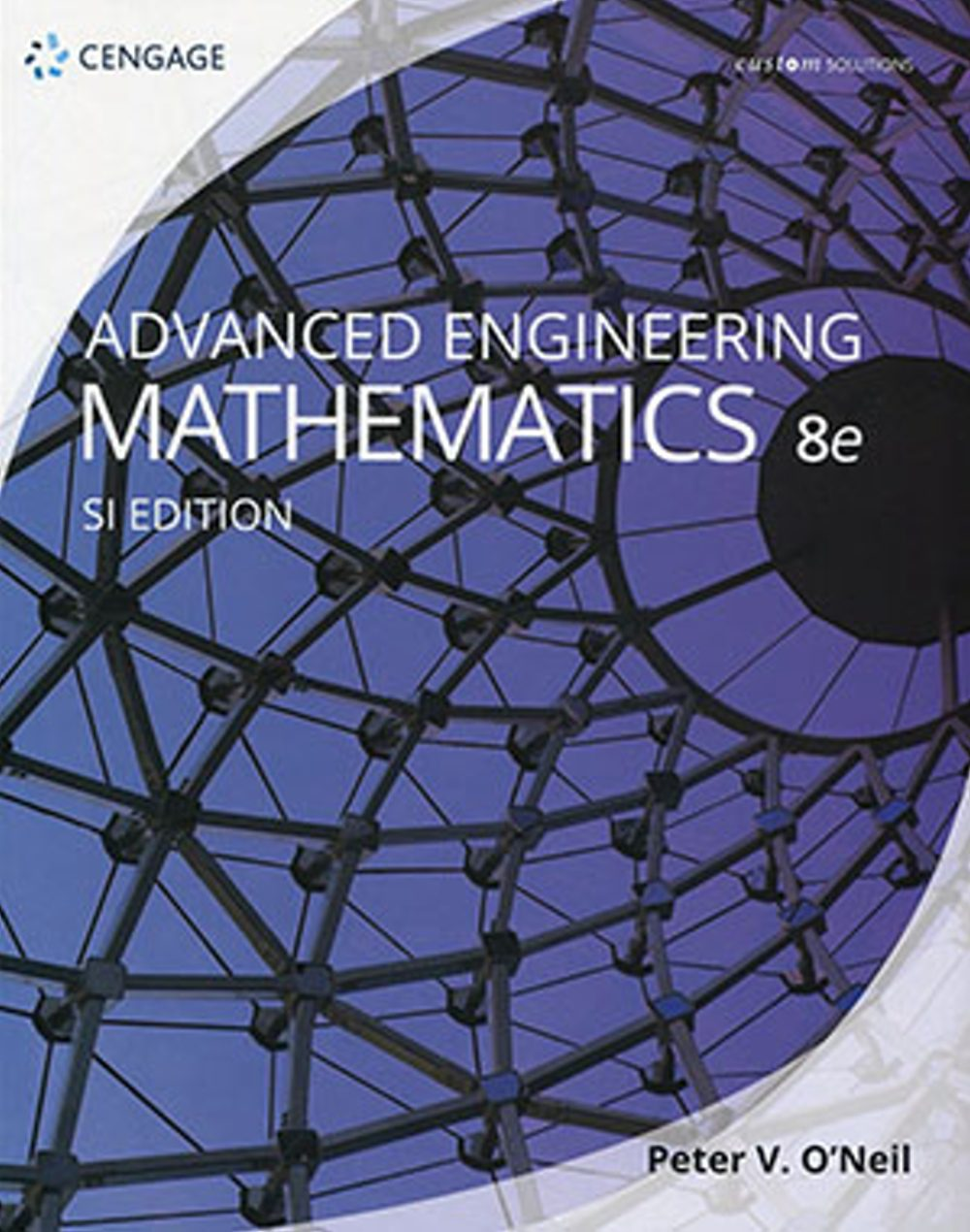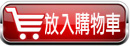沒有庫存 訂購需時10-14天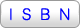9789579282031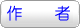Peter V. O’Neil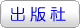華泰文化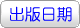2018年3月26日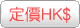473.00  元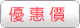HK\$ 449.35省下 \$23.65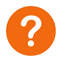ISBN：9789579282031
• 叢書系列：工程數學
• 規格：平裝 / 845頁 / 20 x 25.4 x 2.9 cm / 普通級
工程數學

• 自然科普 > 數學 > 概論

1.Most Course Organizations: Seven distinctive parts clearly focus and differentiate the mathematical ideas and methods while giving you the flexibility to select the sections best suited for your course and student needs.

2.Detailed Examples Illustrate the Use of Notation and the Theory: The numerous examples clarify notation, theory and the underlying computations, followed by the numerical calculations themselves.

3.Specialized and Advanced Topics Added as Web Modules: In order to broaden coverage while keeping the length and cost of the book down, certain topics have been added as convenient web modules rather than appearing in the printed text. These modules include applications of complex analysis to the Dirichlet problem and to inverses of Laplace transforms, Lyapunov functions, the discrete Fourier transform, Maxwell’s equations, numerical methods for solving differential equations, LU factoring of matrices, limit cycles for systems of differential equations, and models of plane fluid flow.

PART I: ORDER DIFFERENTIAL EQUATIONS

Ch 1 First-Order Differential Equations

Ch 2 Second-Order Differential Equations

Ch 3 The Laplace Transform

Ch 4 Series Solutions

PART II: MATRICES AND LINEAR ALGEBRA

Ch 5 Vectors and the Vector Space Rn

Ch 6 Matrices, Determinants, and Linear Systems

Ch 7 Eigenvalues, Diagonalization, and Special Matrices

PART III: SYSTEMS OF DIFFERENTIAL EQUATIONS

Ch 8 Systems of Linear Differential Equations

Ch 9 Nonlinear Systems and Qualitative Analysis

PART IV: VECTOR ANALYSIS

Ch10 Vector Differential Calculus

Ch11 Vector Integral Calculus

PART V: STURM-LIOUVILLE PROBLEMS, FOURIER ANALYSIS AND EIGENFUNCTION EXPANSIONS

Ch12 Sturm-Liouville Problems and Eigenfunction Expansions

Ch13 Fourier Series

Ch14 Fourier Transforms

PART VI: PARTIAL DIFFERENTIAL EQUATIONS

Ch15 The Heat Equation

Ch16 The Wave Equation

Ch17 Laplace’s Equation

Ch18 Special Functions and Applications

Ch19 Transform Methods of Solution

PART VII COMPLEX FUNCTIONS

Ch20 Complex Numbers and Functions

Ch21 Integration

Ch22 Series Representations of Functions

Ch23 Singularities and the Residue Theorem

Ch24 Conformal Mappings

 其 他 著 作 1. 工程數學(8版)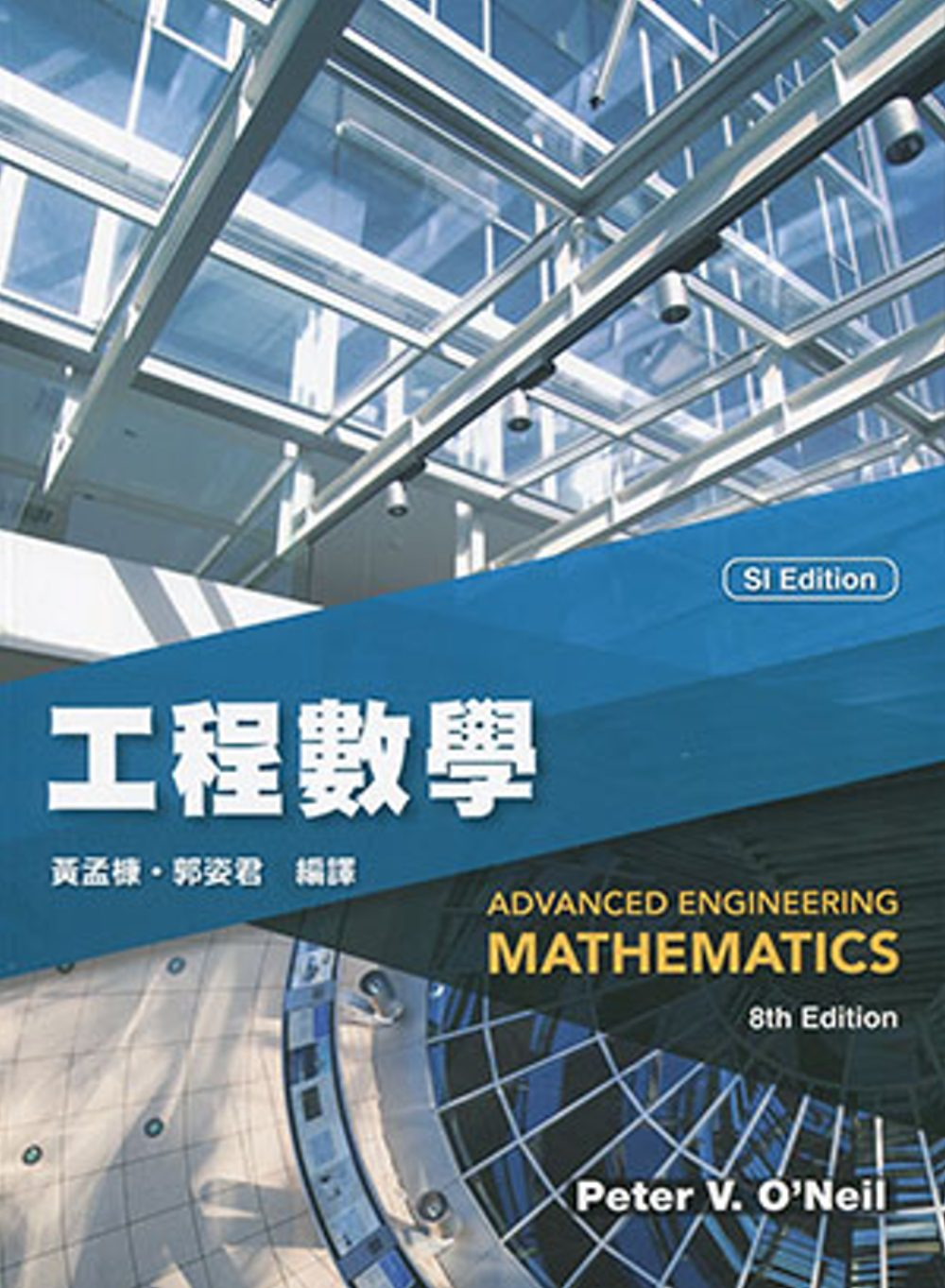2. 高等工程數學(8版)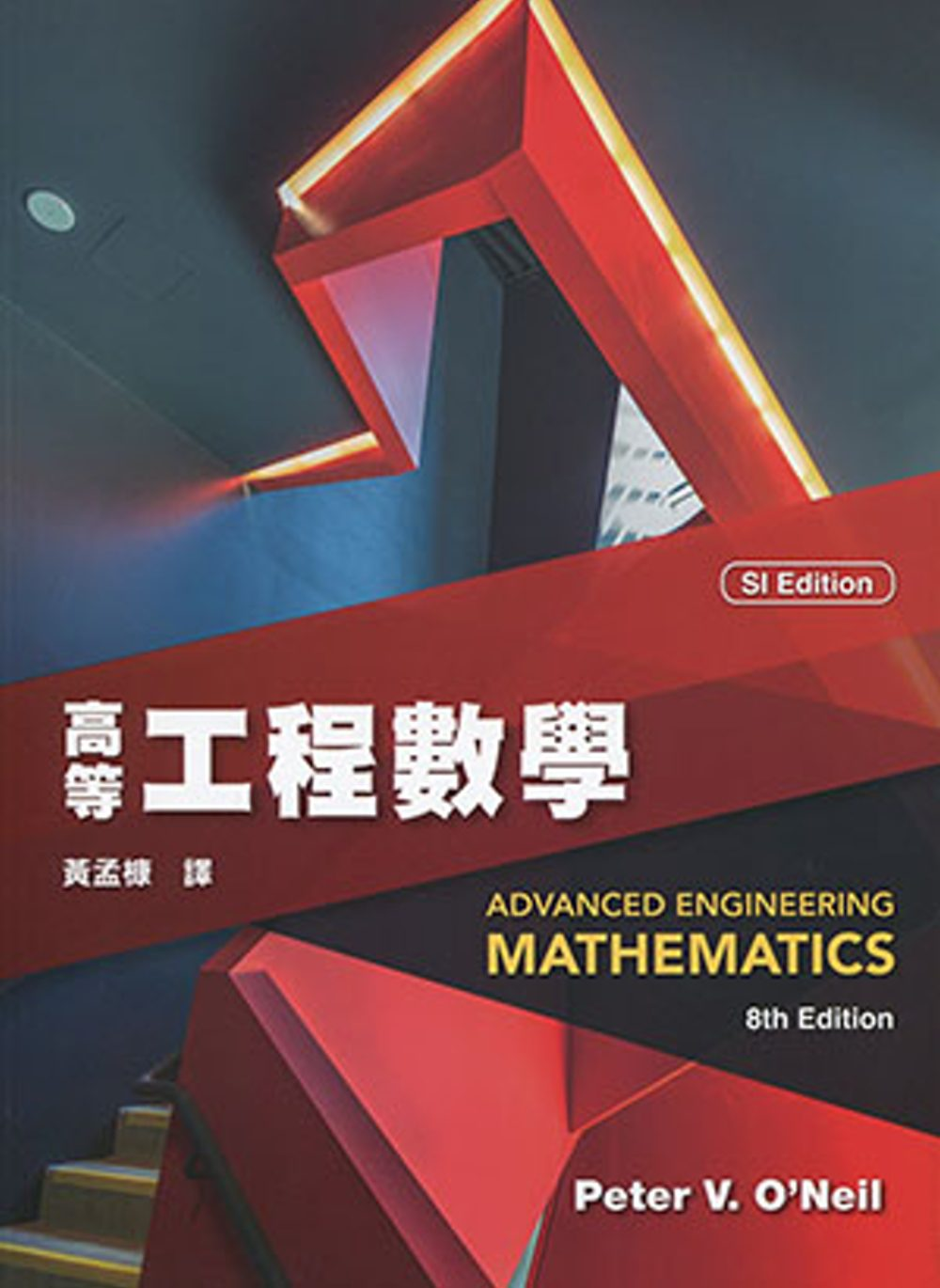3. Advanced Engineering Mathematics(SI Edition)(8版)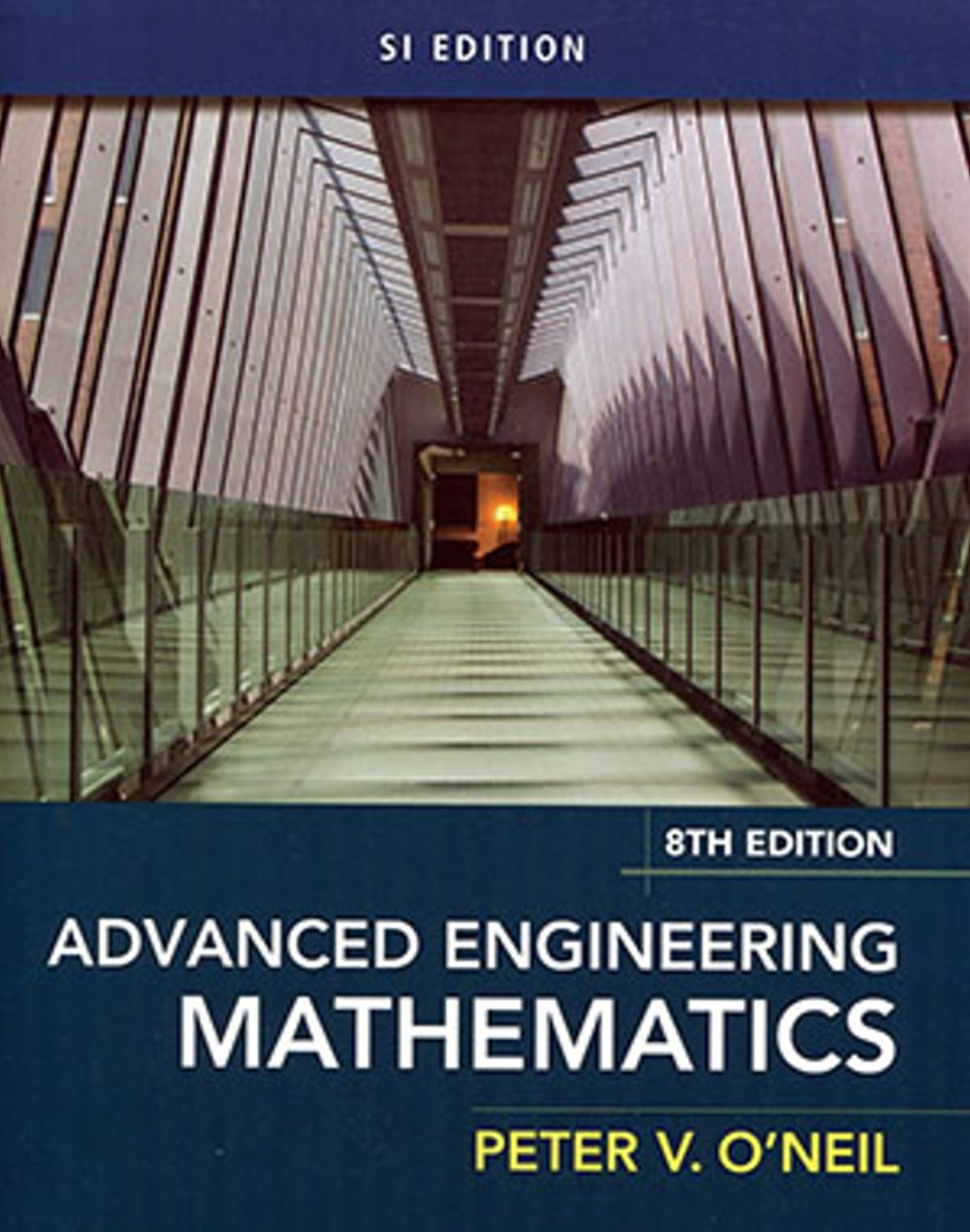4. Advanced Engineering Mathematics (Custom Edition) (bundle Coursemate)7/E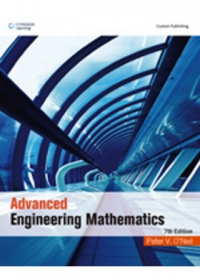5. 高等工程數學(7版) O’Neil/ Advanced Engineering Mathematics 7/e